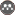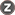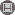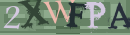Volume 14, Issue 3 (10-2014)                   2014, 14(3): 211-2 | Back to browse issues page

BibTeX | RIS | EndNote | Medlars | ProCite | Reference Manager | RefWorks
Send citation to:Ordokhani Y, Rahimi N. Numerical solution of fractional Volterra integro-differential equations via the rationalized Haar functions. Journal title 2014; 14 (3) :211-2
URL: http://jsci.khu.ac.ir/article-1-1845-en.html
1- Alzahra University
Abstract:   (6939 Views)
In this paper rationalized Haar (RH) functions method is applied to approximate the numerical solution of the fractional Volterra integro-differential equations (FVIDEs). The fractional derivatives are described in Caputo sense. The properties of RH functions are presented, and the operational matrix of the fractional integration together with the product operational matrix are used to reduce the computation of FVIDEs into a system of algebraic equations. By using this technique for solving FVIDEs time and computational are small. Numerical examples are given to demonstrate application of the presented method with RH functions base.In this paper rationalized Haar (RH) functions method is applied to approximate the numerical solution of the fractional Volterra integro-differential equations (FVIDEs). The fractional derivatives are described in Caputo sense. The properties of RH functions are presented, and the operational matrix of the fractional integration together with the product operational matrix are used to reduce the computation of FVIDEs into a system of algebraic equations. By using this technique for solving FVIDEs time and computational are small. Numerical examples are given to demonstrate application of the presented method with RH functions base.
Type of Study: S | Subject: Mathematic
Published: 2014/10/15Rights and permissionsThis work is licensed under a Creative Commons Attribution-NonCommercial 4.0 International License.
 Rights and permissionsThis work is licensed under a Creative Commons Attribution-NonCommercial 4.0 International License.